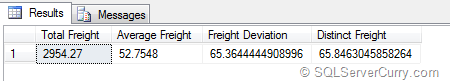### Standard Deviation in SQL Server and Aggregation on Aggregates

SQL Server makes calculation of Standard Deviation relatively easy with the STDEV statistical aggregate function. What I learnt recently was that you can either apply this function to all values or can even select distinct values. You can also perform an aggregation on aggregates that can be used in reports.
I was facing one such requirement where aggregates were to be calculated and we had to consider distinct values as well while calculating standard deviation. Here’s a sample example using the Northwind database.
SELECT
SUM(Freight) as [Total Freight],
AVG(Freight) as [Average Freight],
STDEV(ALL Freight) as [Freight Deviation],
STDEV(DISTINCT Freight) as [Distinct Freight]
FROM Orders
WHERE ShipCountry = 'UK'

As you can see, we are using the ‘ALL’ and ‘DISTINCT’ to specify all or distinct values respectively.If you are creating reports using SSRS, you can create expressions performing aggregation on aggregates. Here’s an example:

=StDev(Sum(Fields!Products.Price))

The StDevP function is also similar except that it performs statistical standard deviation for  all values in the specified expression, it evaluates the ‘entire’ data population. The StDev on the other hand is based on a sample of the data population.